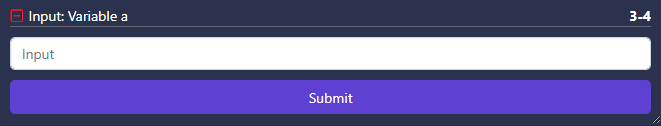# Overview

The first category of Anchors are inputs. These occur in the format: `# @input type`. Input anchors can be used in conjunction with variables to generate an interactive input field in your interface to alter the variable they correspond with.

# All Input Anchors

Anchor

Use

Example

`# @input text`

Numerical input

`# @input number data=a`

`# @input number`

Text input

`# @input text data=a`

# Examples

### Example 1: Example of using an input anchor to prompt an interactive numerical input:

``````a = 3
# @input number data=a
``````

This would prompt the following input card to appear: